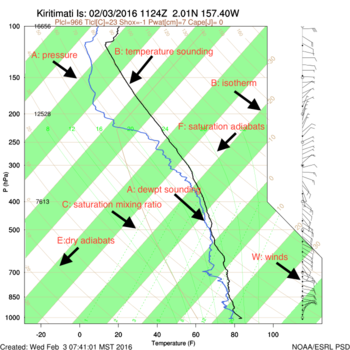## How to read the skewT plots

1. Isobars (P) -- Lines of equal pressure. Solid brown lines that run horizontally from left to right and are labeled on the left side of the diagram. A log scale is used for pressure so spacing isn't equal.
2. Isotherms(T) -- Lines of equal temperature. Straight, solid brown lines that slope from the bottom left to the upper right (thus the name skew) across the diagram. Increments are per degree and are labeled for every 5 degrees in units of Celsius. They are labeled at the bottom of the diagram with corresponding temperatures in Fahrenheit.
3. Dry Adiabats (E) -- Slightly curved, solid brown lines that slant from lower right to upper left. They are labeled every 10 degrees Celsius and indicate the rate of change of temperature in an air parcel of dry air rising or descending adiabatically.
4. Saturation Adiabats (F) -- Slightly curved, solid green lines sloping from lower right to upper left. They are labeled every 2 degrees Celsius and indicate the rate of change of temperature in a saturated air parcel as it rises pseudo-adiabatically. They become parallel to the dry Adiabats at the top of the chart because of the very low moisture content at those levels and stop at 200 mb.
5. Saturation Mixing Ratio Lines (C) -- These are the dashed green, slightly curved lines that slope from the lower left to the upper right that represent lines of equal mixing ratio. They are labeled on the bottom of the diagram in grams per kilogram of water vapor. They extend only to 225 mb and the spacing between them decreases as their values increase.
6. Temperature Curve (B) -- This is the plot of the temperature measurements that were taken from the rawinsonde as it was increasing in height. This curve will always be to the right of the dewpoint curve as you are facing a Skew-T. (Black line)
7. Dewpoint Curve (A) -- This is the plot of the dewpoint measurements increasing with height. This curve will always be to the left of the temperature curve as you are facing a Skew-T. (Blue line).
8. Winds (W) -- Not technically part of a skewT, but the arrows on the right show the speed and direction of the winds for each level.## Terms

1. Dry adiabatic lapse rate - The rate of decrease of temperature with height as an unsaturated parcel of air rises adiabatically (estimated as the lapse rate of the dry adiabat mentioned in the last section). Typically -9.8 ºC/km.
2. Moist adiabatic lapse rate - The rate of decrease of temperature with height as a saturated parcel of air rises pseudo-adiabatically (estimated as the lapse rate of the saturation adiabat mentioned in the last section). Can range from -4 ºC/km to -9 ºC/km but an average value is about -6 ºC/km.
3. Super adiabatic – A condition in which the environmental lapse rate is steeper than the dry adiabats. The most common location for super adiabatic layers is right above the ground on a sunny day.